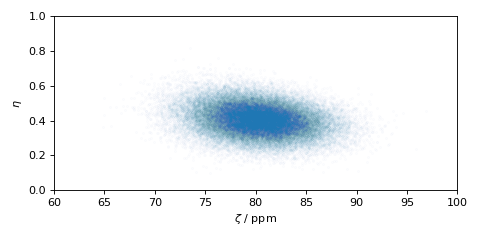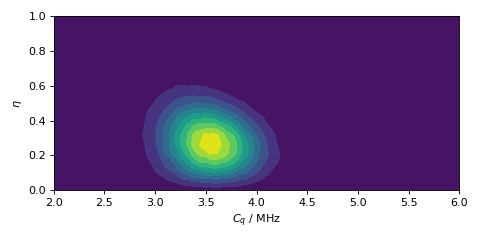# Extended Czjzek distribution¶

The Extended Czjzek distribution models random variations of a second-rank traceless symmetric tensors about a non-zero tensor. See Extended Czjzek distribution and references within for a brief description of the model.

## Extended Czjzek distribution of symmetric shielding tensors¶

To generate an extended Czjzek distribution, use the ExtCzjzekDistribution class as follows.

>>> from mrsimulator.models import ExtCzjzekDistribution
>>> shielding_tensor = {'zeta': 80, 'eta': 0.4}
>>> shielding_model = ExtCzjzekDistribution(shielding_tensor, eps=0.1)


The ExtCzjzekDistribution class accepts two arguments. The first argument is the dominant tensor about which the perturbation applies, and the second parameter, eps, is the perturbation fraction. The minimum value of the eps parameter is 0, which means the distribution is a delta function at the dominant tensor parameters. As the value of eps increases, the distribution gets broader. At eps values greater than 1, the extended Czjzek distribution approaches a Czjzek distribution. In the above example, we create an extended Czjzek distribution about a second-rank traceless symmetric shielding tensor described by anisotropy of 80 ppm and an asymmetry parameter of 0.4. The perturbation fraction is 0.1.

As before, you may either draw random samples from this distribution or generate a probability distribution function. Let’s first draw points from this distribution, using the rvs() method of the instance.

>>> zeta_dist, eta_dist = shielding_model.rvs(size=50000)


In the above example, we draw size=50000 random points of the distribution. The output zeta_dist and eta_dist hold the tensor parameter coordinates of the points, defined in the Haeberlen convention. The scatter plot of these coordinates is shown below.

>>> import matplotlib.pyplot as plt
>>> plt.scatter(zeta_dist, eta_dist, s=4, alpha=0.01)
>>> plt.xlabel('$\zeta$ / ppm')
>>> plt.ylabel('$\eta$')
>>> plt.xlim(60, 100)
>>> plt.ylim(0, 1)
>>> plt.tight_layout()
>>> plt.show()


(png, hires.png, pdf)## Extended Czjzek distribution of symmetric quadrupolar tensors¶

The extended Czjzek distribution of symmetric quadrupolar tensors follows a similar setup as the extended Czjzek distribution of symmetric shielding tensors, shown above. In the following example, we generate the probability distribution function using the pdf() method.

>>> import numpy as np
>>> Cq_range = np.arange(100)*0.04 + 2 # pre-defined Cq range in MHz.
>>> eta_range = np.arange(21)/20  # pre-defined eta range.
...
>>> quad_tensor = {'Cq': 3.5, 'eta': 0.23} # Cq assumed in MHz
>>> Cq, eta, amp = model_quad.pdf(pos=[Cq_range, eta_range])


As with the case with Czjzek distribution, to generate a probability distribution of the extended Czjzek distribution, we need to define a grid system over which the distribution probabilities will be evaluated. We do so by defining the range of coordinates along the two dimensions. In the above example, Cq_range and eta_range are the range of $$\text{Cq}$$ and $$\eta_q$$ coordinates, which is then given as the argument to the pdf() method. The output Cq, eta, and amp hold the two coordinates and amplitude, respectively.

The plot of the extended Czjzek probability distribution is shown below.

>>> import matplotlib.pyplot as plt
>>> plt.contourf(Cq, eta, amp, levels=10)
>>> plt.xlabel('$C_q$ / MHz')
>>> plt.ylabel('$\eta$')
>>> plt.tight_layout()
>>> plt.show()


(png, hires.png, pdf)Note

The pdf method of the instance generates the probability distribution function by first drawing random points from the distribution and then binning it onto a pre-defined grid.